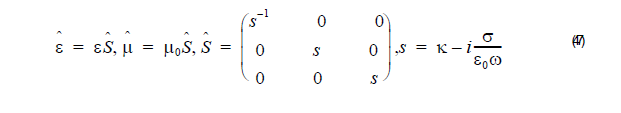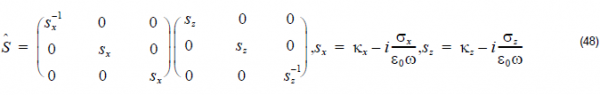The basic FDTD algorithm must be modified at the boundaries of the computational
window where suitable numerical absorbing boundary conditions (ABC) are applied.
This is one of the most challenging parts of FDTD simulations. There are several
choices for the type of boundary conditions. The Perfectly Matched Layer (PML)
boundary conditions have the best performance. Our FDTD simulator uses the
Anisotropic PML, or so-called Un-split PML (UPML) version. The theory of the UPML
is very well explained in some of the references given here. The UPML boundary
conditions are physical rather than numerical because their implementation is based
on a Maxwellian formulation rather than on a mathematical model. Their absorbing
properties are physically equivalent to the properties of an absorbing uni-axial
anisotropic medium with the following permittivity and permeability tensors:A plane wave incident on a half space composed of the above uniaxial medium with
an interface in the x = const plane is purely transmitted into it. The reflectionless
property is completely independent of the angle of incidence, polarization and
frequency of the incident wave. The numerical implementation of the UPML in a 2D
(X-Z) computational window requires the introduction of such perfectly matched
absorbing layers on all the sides. The corner regions need special attention. In these
regions the tensor from Equation 47 must be modified to:The minimization of the numerical reflectance of the Anisotropic PML layers requires
spatial scaling of the conductivity profile from zero (at the interface of the PML) to a
maximum value at the end of the computational window:where L is the thickness of the Anisotropic PML. Typical values for the parameter m
are between 2 and 4.